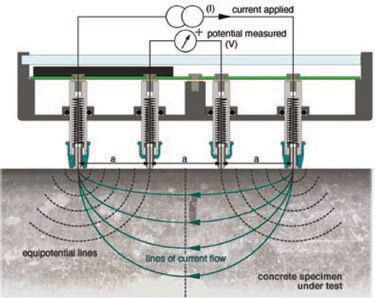Homepage » Knowledge Centre » NDT » Electrical Resistivity of Concrete

# Electrical Resistivity of Concrete

The use of electrical resistivity method for quality control and evaluation of concrete durability is becoming very popular among scholars and engineers. The measurement of electrical resistivity of concrete provides useful information about the microstructure of concrete material. The test procedure has since been standardized by AASHTO TP 95. This method is adapted by several Department of Transportation (DOTs) to replace the labour-intensive and time-consuming methods such as the ASTM C 1202, “Electrical Indication of Concrete’s Ability to Resist Chloride Ion Penetration”, generally known as the Rapid Chloride Peremeabiliy Test (RCPT).

## What is Electrical Resistivity

Electrical resistivity, ρ, is an inherent characteristic of concrete material, which is independent of the geometry of the test sample. Resistivity is calculated through measuring electrical resistance, R,  in an appropriately designed circuit, and applying certain geometry factor, γ. Equation 1 provides a simple representation of such relationship.

Equation 1:            ρ=γ.R

The resistance of concrete against electrical current can be correlated to its resistance against chloride ion movement. Concrete durability depends to the properties of its microstructure, such as  the pore size distribution and the shape of the interconnections (Layssi et al, 2015). Generally, a dense microstructure provides better durability compared to porous micorstructure. The very concept behind most electrical resistivity techniques is to somehow evaluate the electrical properties (resistance in this case) of the microstructure of concrete.

## Electrical Resistivity of Concrete

As discussed earlier, resistivity is determined by measuring electrical resistance and applying appropriate geometry factors. Measuring the resistance is more difficult than it looks, mainly because concrete is a porous material, and depending on the degree of saturation, its electrical properties changes from insulator (dry concrete), to conductive (saturated concrete). The other reason contributing to the complexity of the measurements is the capacitance properties of concrete when it is wet. Researchers have developed and studied different test techniques. Two formats of the test has become very popular:

### Four-probe (electrode) Method

Four-probe method uses four electrode to measure the surface electrical resistivity of concrete. The arrangement of the electrodes is famously known as Wenner Array, and consists of four electrodes that are placed over the surface of concrete. The outer electrodes create an electrical current in the concrete, and the interior electrodes measure the induced potential. The resistance is then calculated by dividing the potential difference to the current.  Th Wenner probe is the most famous of the two, mainly because of its simplicity, and being portable, which make it great for field test.Photo adapted from Proceq resipod user manual

### Two-probe (electrode) Method

Two-probe method is used to measure the bulk electrical resistivity of concrete. In this technique, the concrete sample is placed between the two electrodes, and the potential is measured after applying the current. Normally, a wet sponge is used to provide contact between the electrode plates and concrete surface. Since the current is passing through the bulk of concrete, this technique provides better indication of concrete electrical resistance.

## Application of Electrical Resistivity Methods for Concrete

The electrical resistivity of concrete is well correlated with some important performance characteristics of concrete such as

+ Water absorption

+ Chloride diffusion coefficient

+ Corrosion rate of embedded steel.

Electrical resistivity measurement have proved to be a very suitable method for investigating the properties of fresh concrete such as setting time, moisture content, etc. Nadelman and Kurtis (2014) demonstrated the use of surface resistivity method for the influence of binder composition on the rate of microstructural development in concrete, which makes it an alternative for assessing future performance.

What Influences the Measurements?

With all the advantages the electrical resistivity methods offer, they have some limitations and restrictions. The electrical resistivity of concrete might be affected by the following parameters:

+ Pore size distribution

+ Pore interconnection

+ Conductivity of pore fluid

+ Degree of saturation

+ Temperature variation

## 5 thoughts on “Electrical Resistivity of Concrete”

1.How can we measure resistivity of concrete cube with embedded four probe method

2.Very useful thanks a lot

3.Is it correct if I use ASTM C1760 (for measuring bulk electrical conductivity of hardened concrete) to determine electrical resistivity from the current measured and applied voltage.